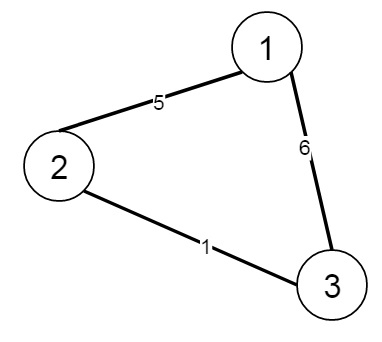# Connecting Cities With Minimum Cost in Pythonn

Suppose there are N cities numbered from 1 to N. We have connections, where each connection [i] is [city1, city2, cost] this represents the cost to connect city1 and city2 together. We have to find the minimum cost so that for every pair of cities, there exists a path of connections (possibly of length 1) that connects those two cities together. The cost is the sum of the connection costs used. If the task is impossible, return -1.

So if the graph is like −Then the output will be 6, Choosing any two cities will connect all cities, so we choose the minimum 2, [1, 5]

To solve this, we will follow these steps −

• Define method called find(), this will take x

• if parent[x] is -1, then return x

• parent[x] := find(parent[x])

• return parent[x]

• Make another method called union(), this will take x and y

• parent_x := find(x), parent_y := find(y)

• if parent_x = parent_y, then return, otherwise parent[parent_y] := parent_x

• From the main method, it will take n and conn

• parent := an array of size n and fill this with – 1, set disjoint := n – 1, cost := 0

• c := sorted list of conn, sort this based on the cost

• i := 0

• while i < length of c and disjoint is not 0

• x := c[i, 0] and y := c[i, 1]

• if find(x) is not same as find(y), then decrease disjoint by 1, increase the cost by c[i, 2], and perform union(x, y)

• increase i by 1

• return cost when disjoint is 0, otherwise return -1

## Example(Python)

Let us see the following implementation to get a better understanding −

Live Demo

class Solution(object):
def find(self, x):
if self.parent[x] == -1:
return x
self.parent[x] = self.find(self.parent[x])
return self.parent[x]
def union(self,x,y):
parent_x = self.find(x)
parent_y = self.find(y)
if parent_x == parent_y:
return
self.parent[parent_y] = parent_x
def minimumCost(self, n, connections):
self.parent = [ -1 for i in range(n+1)]
disjoint = n-1
cost = 0
c = sorted(connections,key=lambda v:v)
i = 0
while i<len(c) and disjoint:
x = c[i]
y = c[i]
if self.find(x)!=self.find(y):
disjoint-=1
cost+=c[i]
self.union(x,y)
i+=1
return cost if not disjoint else -1
ob = Solution()
print(ob.minimumCost(3, [[1,2,5], [1,3,6], [2,3,1]]))

## Input

3
[[1,2,5],[1,3,6],[2,3,1]]

## Output

-1